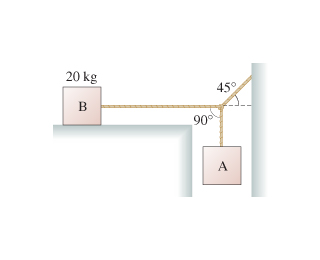# Problem: Block B in the figure(Figure 1) rests on a surface for which the static and kinetic coefficients of friction are 0.63 and 0.40, respectively. The ropes are massless.What is the maximum mass of block A for which the system remains in static equilibrium?Express your answer to two significant figures and include the appropriate units.

###### FREE Expert Solution

Static frictional force by B = T

T = μsmg

The force balance weight of block A.

93% (57 ratings)###### Problem Details

Block B in the figure(Figure 1) rests on a surface for which the static and kinetic coefficients of friction are 0.63 and 0.40, respectively. The ropes are massless.What is the maximum mass of block A for which the system remains in static equilibrium?

Express your answer to two significant figures and include the appropriate units.

Frequently Asked Questions

What scientific concept do you need to know in order to solve this problem?

Our tutors have indicated that to solve this problem you will need to apply the Systems of Objects with Friction concept. You can view video lessons to learn Systems of Objects with Friction. Or if you need more Systems of Objects with Friction practice, you can also practice Systems of Objects with Friction practice problems.

What professor is this problem relevant for?

Based on our data, we think this problem is relevant for Professor Demchenko's class at VCU.